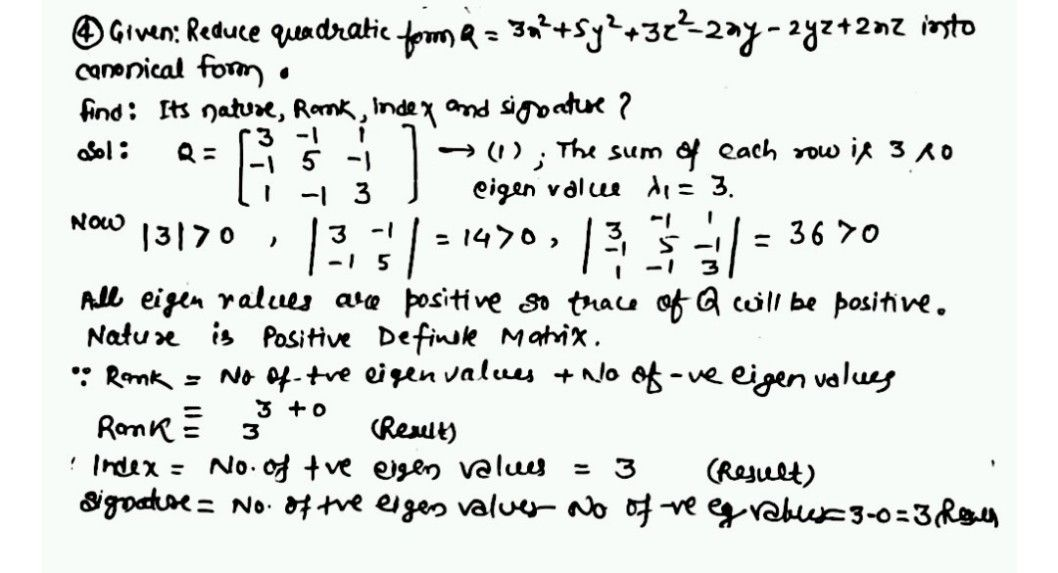Symbol
Problem$\bar{1}$ $0m$ $1$ $0n$ $v$ $m-11$ $1$ $1$ 1 $v$ $2^{\right)}$ $4.$ Reduce the quadratic form $Q=3x^{2}+5y^{2}+3x^{2}-2xy-2yz+2xz$ to canonical form and hence find its nature, rank, index and signature. $5$ Reduce the quadratic form $0-x$ $2+2\times 2$ to canonical form and bence find its nature
Calculus
Search count: 128
SolutionQanda teacher - Arushithnks for asking good questions
Please evaluate:- 1. Click on top right corner EVALUATE button. 2.Select 5 star. 3.Click on gift coins + three times(C3000)(Not reduce your coins). 4.Click on DONE button on top right corner.
read ? read ? and follow its free for students